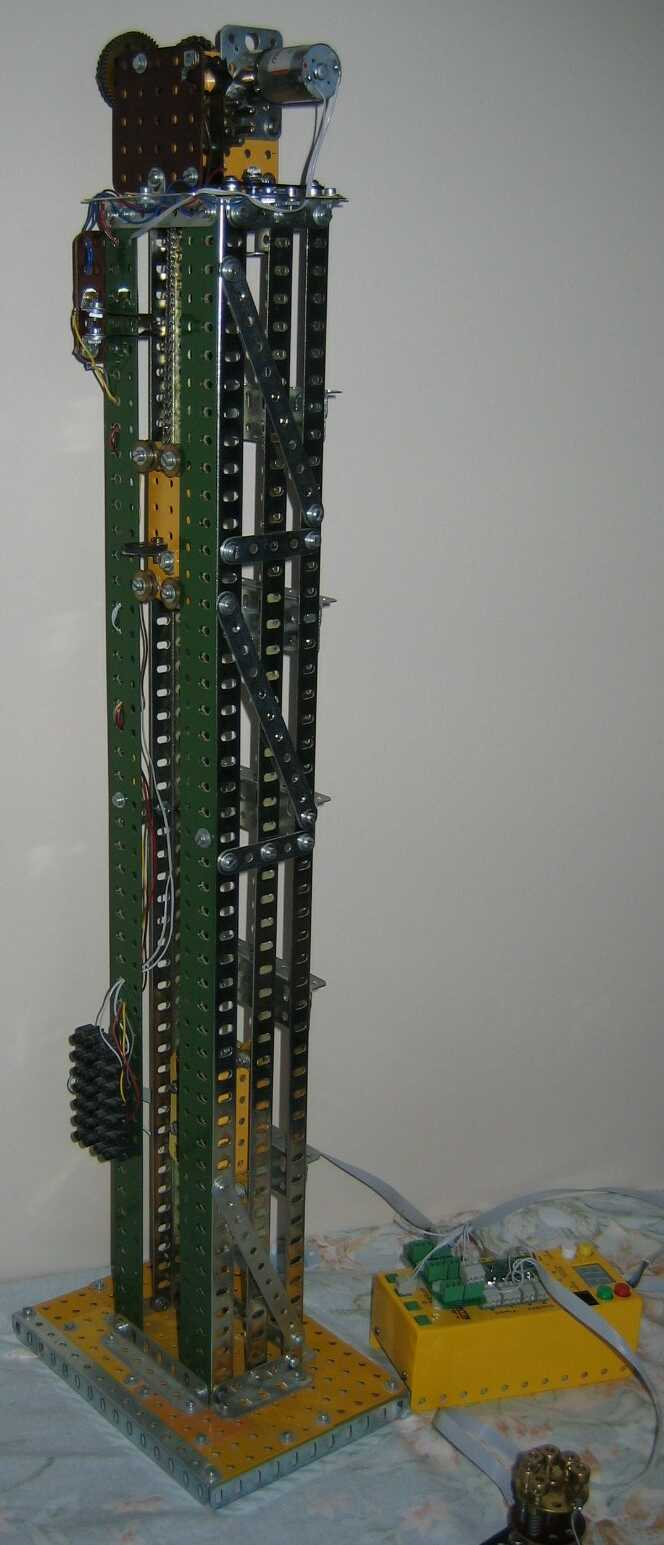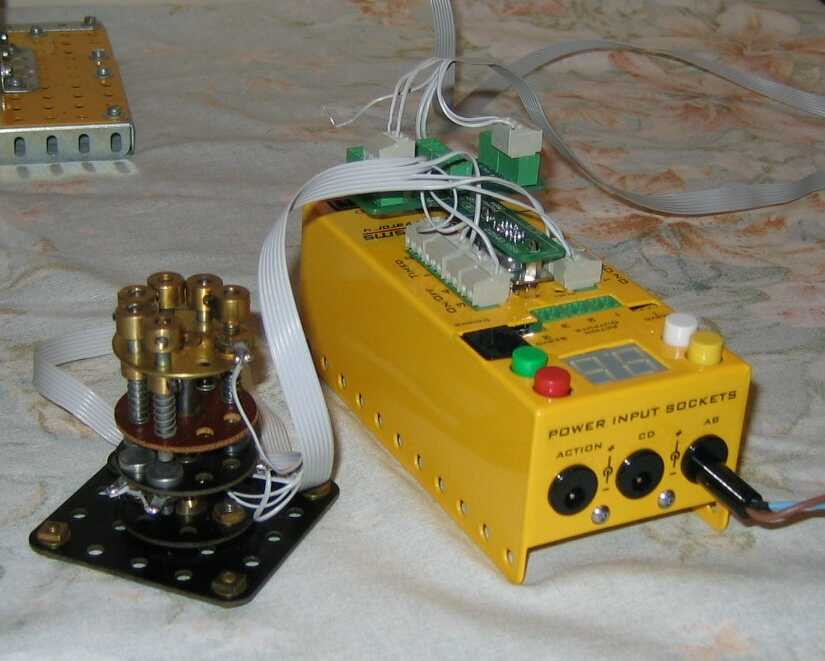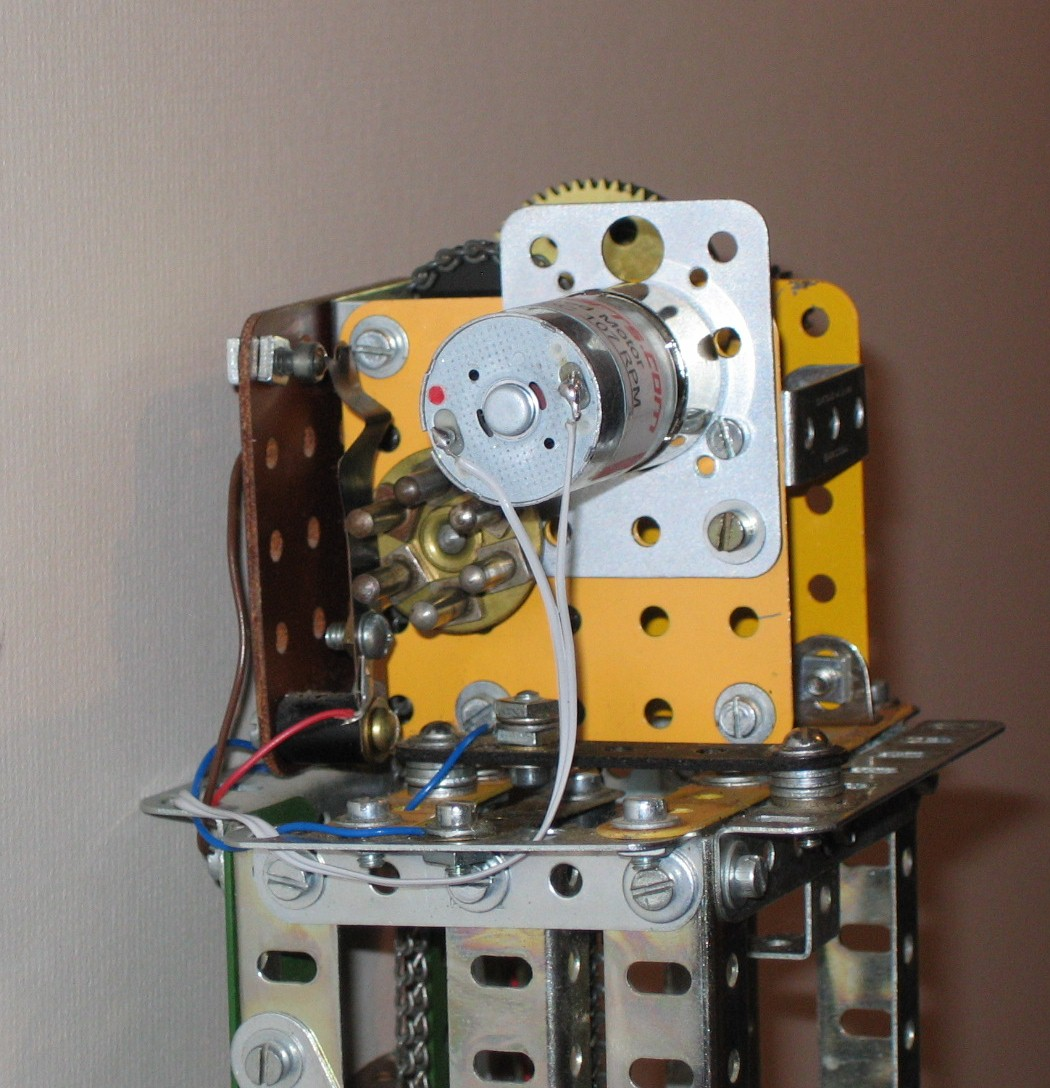# An Update on Keith Cameron's Lift

The Model is based on a design by Keith Cameron published in Constructor quartely No 7 march 1990 and is a simple example of the application of the Motorvator. This really complements my tram model as in the latter case this was an example of how the Motorvator could considerably simplify the automatic operation of a model without user intervention, whereas the lift model demonstrates how the Motorvator can considerably simplify a model requiring user intervention. I have seen a lift model wth a very complex circuit involving numerous relays to do the same job.The model is accurate at floor levels to about +- 3mm max.and more usually to +- 2mm. It might be possible to improve on this with further tweaking. The problems are motor overrun , positioning of the rotation counter when it stops and possibly the rotation counter missing a pulse ( I don't think this happens although it might). I have used a Meccanisms 107rpm motor which seems just right for the job. Of course a stepper would give exact positioning and I might modify the model to test this.On the model, selecting ground or 5th floor will re register the position and can always be selected if a sgnificant position error is noticed although in practice I have rarely found an error greater than 4mm. Andrew has suggested that greater accuracy might be obtained by slowing the motor to reduce overun as the lift approaches floor level and I am experimenting with this.

Note that the limit switch in the photo IS the ground floor switch: it is triggered by the counterweight which is of course at the top when the lift car is at the bottom!The Consul of lift call buttons is made of standard Meccano and Electrikit parts. the connection to the motorvator is to OnOff 1(two wires), OnOff 2 to 4 (+ive pin only), Timed 1 (centre pin only) and timed 2 (centre pin only). The ground is connected at the consul to all 6 inputs.The inputs from the model are the rotation counter connected by two wires to Timed 4 (ground and centre signal pins), the limit switch for ground and fifth (two switches one connection to timed 3) and the motor connected to motor port B.I have used the Director socket extension board but I imagine that the Analogue inputs on the Motorvator could be used instead with a small change in the program.

As you can see the program consists of a simple loop which tests for a button press for each floor in turn.

The Consul could be considered as a model specific "Director" and the idea could be extended to much more complex models.

Here is the code for the MotorVator

```Begin Program Declare Byte Counter=0 Declare Byte Totalcount=0 Declare Byte Stoptb=0 Declare Byte Ground=0 Declare Byte First=0 Declare Byte Second=0 Declare Byte Third=0 Declare Byte Fourth=0 declare Byte Fifth=0 Declare Byte DiffCount=0 Declare Byte StartCount=0 Declare Byte Finalcount=0 Declare Byte AtFloor=0 Declare Byte Speed=50 Disable Events SetEvent 19,rotationcount Enable Events Call Initialise()  ;This initialises the program and takes the lift to the Ground floor Loop     ground=readOnOff(1)         if ground=1 then         AtFloor=0     ;This variable prevents any action if the lift is already at the floor for the button pressed         Call Initialise()  ;also reregisters position     end if     first=readOnOff(2)     if AtFloor<>1 then     if first=1 then         call  GoToFirst()         Atfloor=1     end if     end if     second=readOnOff(3)     if second=1 then     if AtFloor<>2 then         Call GoToSecond()         AtFloor=2     end if     end if     third=readOnOff(4)     if third=1 then     if AtFloor<>3 then             Call GoToThird()         AtFloor=3     end if     end if     fourth=readtimedstate(1)     if fourth=1 then     if AtFloor<>4 then             Call GoToFourth()                 AtFloor=4     end if     end if     fifth=Readtimedstate(2)     if fifth=1 then         Call GoToFifth()     ;Also reregisters position         AtFloor=5     end if End Loop      End Program Declare EventHandler RotationCount()     counter=ReadTimedState(4)     If counter=1 then         TotalCount=TotalCount+1     End  If     DisplayNumber Totalcount End EventHandler Declare Function Initialise()     SetMotor 2,backward,speed     Do         stoptb=readtimedstate(3)     Until stoptb=1     Do         SetMotor 2,backward,speed         counter=ReadTimedState(4)     Until counter=0     Stopmotors     totalcount=0 End Function Declare Function GoToFifth()     SetMotor 2,Forward,speed     Do     Stoptb=readtimedstate(3)     Until Stoptb=1     ;displayNumber totalcount     Do         counter=1                     ; An attempt to move the counter to a standard position         displayNumber counter         SetMotor 2,forward,speed         counter=ReadTimedState(4)         displayNumber counter     Until counter=0     Stopmotors     totalcount=120     stoptb=0 End Function Declare Function GoToFirst()     If TotalCount<=24 then     Do         SetMotor 2, forward,speed     Until totalcount=26     totalcount=24     End If     If TotalCount>24 then     StartCount=totalcount     Do         SetMotor 2, backward,speed         DiffCount=Startcount-totalcount  ;calculates the position relative to the last count         finalcount=Startcount+DiffCount         Until finalcount=30     TotalCount=24     End If     StopMotors End Function Declare Function GoToSecond()     If TotalCount<=48 then     Do         SetMotor 2, forward,speed     Until totalcount=48     End If     If TotalCount>48 then     StartCount=totalcount     Do         SetMotor 2, backward,speed         DiffCount=Startcount-totalcount         finalcount=Startcount+DiffCount     Until finalcount=53   ;In an ideal world this would be 48 but changes were made to allow for motor overun.      TotalCount=48     End If     StopMotors End Function Declare Function GoToThird()     If TotalCount<=72 then     Do         SetMotor 2, forward,speed     Until totalcount=72     End If     If TotalCount>72 then     StartCount=totalcount     Do         SetMotor 2, backward,speed         DiffCount=Startcount-totalcount         finalcount=Startcount+DiffCount     Until finalcount=76        ;see note above     Totalcount=72     End If     StopMotors End Function Declare Function GoToFourth()     If TotalCount<=96 then     Do         SetMotor 2, forward,speed     Until totalcount=96     End If     If TotalCount>96 then     StartCount=totalcount     Do         SetMotor 2, backward,speed         DiffCount=Startcount-totalcount         finalcount=Startcount+DiffCount         DisplayNumber finalcount         Until finalcount=101     End If     TotalCount=96     StopMotors End Function ```